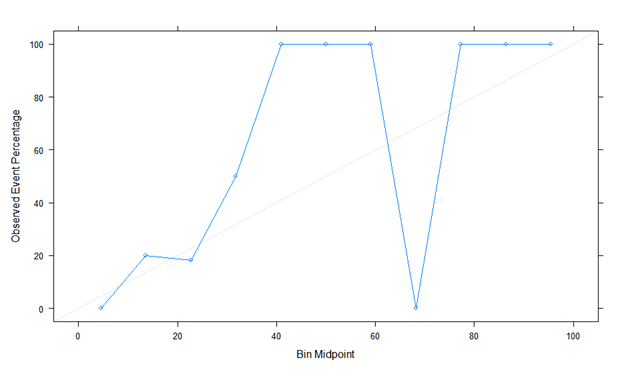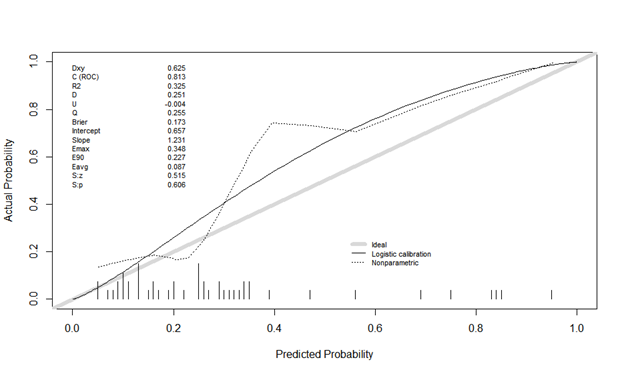# Calibration plots

Hi! I need to ask about calibration again. I created the LR model and did a calibration plot on the test data (n=49). Below are two variants:
Here is the first calibration plot using Caret. Looks like a true story!The second is calibration plot using Rms, val.prob (predicted probability, class). The actual probability is odd, and it looks different. Is there something I’m doing wrong?Please post this as a reply at the end of RMS Describing, Resampling, Validating, and Simplifying the Model and I’ll remove it here. Note that binning is not valid in this context so re-do your own calibration curve without binning before posting. If you are unable to do this, re-post it as-is.

1 Like